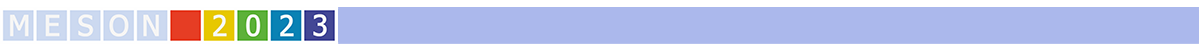#MESON2023

Jun 22 – 27, 2023
Auditorium Maximum
Europe/Warsaw timezone

## Molecular-type pentaquarks from coupled channel dynamics with strangeness S=-2

Jun 22, 2023, 4:50 PM
25m
Medium lecture hall (A) (Auditorium Maximum)

Invited parallel

### Speaker

Volodymyr Magas (University of Barcelona)

### Description

The existence of the nucleonic pentaquark resonances $P_c(4312)$, $P_c(4380)$, $P_c(4440)$ and $P_c(4457)$, established by the LHCb collaboration, has been one of the major discoveries in hadron physics in the latest years. This has been followed by the discovery of pentaquarks with one unit of strangeness, $P_{cs}(4338)$ and $P_{cs}(4457)$. Most of these states can be understood as hadronic molecules, namely bound states of a sufficiently attractive meson-baryon interaction.

In our recent work  we revisit the procedure of the unitarized coupled-channel hidden gauge formalism, which has been a very successful approach in explaining other exotic hadrons in the charm and hidden charm sectors. Employing realistic regularization parameters, we predict double strangeness pentaquarks of molecular nature . The first one, $\Xi(4493)$, is a $J^P=1/2^-$ resonance generated from the interaction of pseudoscalar mesons with baryons, which couples most strongly to the $\bar{D}\Omega_c$ and $\bar{D}_s\Xi_c^\prime$ channels and can be most likely observed via its decay to $\eta_c \Xi$ states, for instance in decay processes of bottomed baryons, such as $\Xi_b \to \Xi\ \eta_c\ \phi$ and $\Omega_b \to \Xi\ \eta_c\ {\bar K}$. The other one, $\Xi(4633)$, is a spin-degenerate resonance which can have $J^P=1/2^-$ or $J^P= 3/2^-$ and is obtained from the interaction of vector mesons with baryons. It couples dominantly to the $\bar{D}^*\Omega_c$ and $\bar{D}_s^*\Xi_c^\prime$ channels and it should be looked for in invariant mass spectra of $J/\Psi\, \Xi$ pairs which could be produced in the decays $\Xi_b \to \Xi\ J/\Psi\ \phi$ and $\Omega_b \to \Xi\ J/\Psi\ {\bar K}$.

These molecular-type pentaquarks are dynamically generated in a very specific and unique way, via a strong non-diagonal attraction between the two heaviest meson-baryon channels .
This effect was overlooked before because the very mild attraction in the diagonal interaction of the heaviest meson-baryon state was neglected, hence effectively preventing the coupled-channel mechanism to take effect.

We hope that our work would stimulate experiments looking for these type of pentaquarks, the discovery of which would enrich the family of their already observed $S=0$ and $S=-1$ pentaquark partners.

 J. A. Marse-Valera, V. K. Magas and A. Ramos, Phys. Rev. Lett. 130 (2023) no.9, 9.

### Primary authors

Angels Ramos (University of Barcelona) Arnau Marsé-Valera (University of Barcelona) Volodymyr Magas (University of Barcelona)